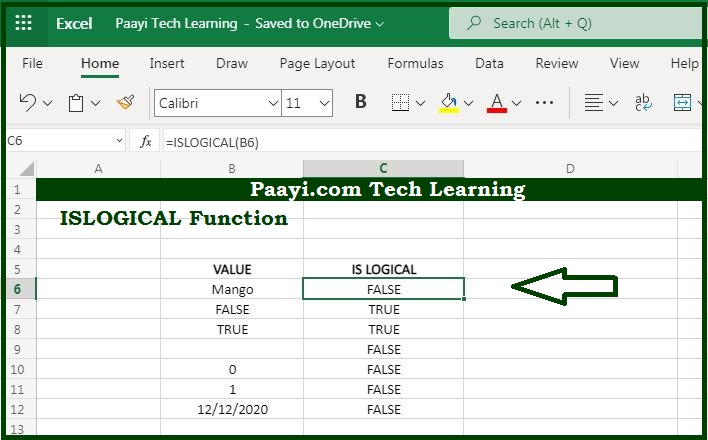# Learn How to Use Microsoft Excel ISLOGICAL Function

Written by | 0 Comments | 705 Views

In this article, you will learn how to use the Microsoft Excel ISLOGICAL function and its prime function in Microsoft Excel. You will also get to know the Microsoft Excel ISLOGICAL function return value and syntax with the help of some examples.

Microsoft Excel ISLOGICAL Function

The main function of the Microsoft Excel ISLOGICAL function is to test if the value is logical or not. That means with the help of ISLOGICAL function you can able to get the return value as the TRUE if the cell contains the logical values and FLASE if the cell does not have logical values or any other value including an empty cell.

Return Value of ISLOGICAL Function

The return value will be logical TRUE or FALSE.

Syntax of ISLOGICAL Function

=ISLOGICAL(value)

Where the arguments:

• reference: It is the value to test as logical.

## How to Use Microsoft Excel ISLOGICAL Function?You can use the ISLOGICAL function to check whether the cell contains any logical value or not. With ISLOGICAL function you can able to get the return value as the TRUE if there is a logical value and FALSE if there is not any logical value in the cell. It should be noted that 1 and 0 are not evaluated as TRUE or FALSE. But, you can use the AND function to evaluate 1 and 0.